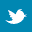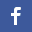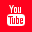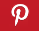Completely Solved C, C++ Programs Assignment.Quick Search Database Videos Tutorials Ebooks Forums FAQ Aboutus Household Industrial Manufacturing Service Shopping Transportation### c++ program to implement a Square Matrix Class

 Filed Under:

Problem Statement :
Design and implement a SquareMatrix class. There should be appropriate constructors and destructor. Also implement following operations appropriately with overloaded operators :
&#61548; >> And << to input and output matrices into appropriate format.
&#61548; Subtraction of two matrices.
&#61548; Multiplication of a matrix by a scalar.
&#61548; Multiplication of two matrices
&#61548; Transposition of a matrix. (Hint: The matrix AT of order n by m obtained by interchanging rows and columns in a matrix A of order m by n is called the transpose of A.)

Logical Approach :
The above program is done using an Abstract Data Type (ADT) called class. A class is a mechanism to create objects. In this program a class named sqMatrix is created.
It contains the following data members :
&#61548; **matrix
&#61548; dim(dimension)
It contains the following member functions :

&#61548; sqMatrix(int=5)-Parameterized constructor, used to initialize a square matrix.
&#61548; sqMatrix(const sqMatrix&)-Copy constructor, used to make a new copy of a square matrix.
&#61548; ~sqMatrix()-Destructor.
&#61548; operator+(const sqMatrix&)-Used to add two square matrices.
&#61548; operator-(const sqMatrix&)-Used to subtract two square matrices.
&#61548; operator*(const sqMatrix&)-Used to multiply two square matrices.
&#61548; operator*(int)-Used to multiply a square matrix with a scalar value.
&#61548; sqMatrix operator^(const sqMatrix&)-Used to get transpose of a square matrix.
&#61548; istream& operator>>(istream&,sqMatrix&)-Overload >>(cin) operator which takes a square matrix as input.
&#61548; ostream& operator<<(ostream&,sqMatrix)-/Overload <<(cout) operator which displays a square matrix.

In this program we have used operator overloading feature. Operator overloading (less commonly known as operator ad-hoc polymorphism) is a specific case of polymorphism in which some or all of operators like +, =, or == have different implementations depending on the types of their arguments. Sometimes the overloadings are defined by the language; sometimes the programmer can implement support for new types.
To define an additional task to an operator,we must specify what it means in relation to the class to which the operator is applied. This is done with help of an operator function,which describes the task. Operator functions must be either member functions(non-static) or friend functions. In this program,we have done all the operations by using member functions except overloading of << and >> operators. These two operators are overloaded using friend functions.
User should give dimension of the square matrix as input and then the original matrix with which operations are going to be performed.

Algorithm :
Creating an ADT with name sqMatrix

Step 2:Declaring private data members
int **matrix//Declaring an integer double pointer
int dim//Declaring an integer
Step 3:Declaring public member functions
sqMatrix(int=5);
sqMatrix(const sqMatrix&);
~sqMatrix();
operator+(const sqMatrix&);
operator-(const sqMatrix&);
operator*(int);
operator*(const sqMatrix&);
operator^(const sqMatrix&);
operator>>(istream&,sqMatrix&);
operator<<(ostream&,sqMatrix&);
};

Parameterized constructor

sqMatrix(int dimension)
{
dynamically instantiate sqMatrix with dimension passed as parameter;
for( i=0;i<dim;i++)
{
for(j=0;j<dim;j++)
matrix[i][j]=0;
}
dim=dimension;
}

Destructor

~sqMatrix()
{
for(i=0;i<dim;i++)
{
deallocate matrix[i];
}
}

Copy constructor

sqMatrix(const sqMatrix &mat)
{
dynamically instantiate memory for a new cop of the square matrix;
for(i=0;i<mat.dim;i++)
{
for(j=0;j<mat.dim;j++)
sqMatrix::matrix[i][j]=mat.matrix[i][j];
}
dim=mat.dim;
}

This function is used to add two square matrices

Procedure operator+(const sqMatrix &mat)
{
sqMatrix tmp(mat.dim);
for( i=0;i<mat.dim;i++)
{
for(j=0;j<mat.dim;j++)
{
tmp.matrix[i][j]=matrix[i][j]+mat.matrix[i][j];
}
}
}

This function is used to subtract two square matrices

Procedure operator-(const sqMatrix &mat)
{
sqMatrix tmp(mat.dim);
for( i=0;i<mat.dim;i++)
{
for(j=0;j<mat.dim;j++)
{
tmp.matrix[i][j]=matrix[i][j]-mat.matrix[i][j];
}
}
}

This function is used to multiply two square matrices

Procedure operator*(const sqMatrix &mat)
{
sqMatrix tmp(dim);
for(i=0;i<dim;i++)
{
for(j=0;j<dim;j++)
{
tmp.matrix[i][j]=0;
for(k=0;k<dim;k++)
{
tmp.matrix[i][j]=tmp.matrix[i][j]+matrix[i][k]*mat.matrix[k][j];
}
}
}
}

This function is used to multiply a square matrix with a scalar value

Procedure operator*(int c)
{
sqMatrix tmp(dim);
for(i=0;i<dim;i++)
{
for(j=0;j<dim;j++)
tmp.matrix[i][j] = matrix[i][j] * c;
}
}

This function is used to get transpose of a square matrix

Procedure operator^(const sqMatrix &mat)
{
for( i=0;i<dim;i++)
{
for(j=0;j<dim;j++)
mat.matrix[i][j]=matrix[j][i];
}
}

Overload <<(cout) operator which displays a square matrix

Procedure operator<<(ostream &s,sqMatrix mat)
{
for(i=0;i<mat.dim;i++)
{
for(j=0;j<mat.dim;j++)
s<<mat.matrix[i][j];
}
}

Overload >>(cin) operator which takes a square matrix as input

Procedure operator>>(istream &s,sqMatrix &mat)
{
for( i=0;i<mat.dim;i++)
{
for(j=0;j<mat.dim;j++)
s>>mat.matrix[i][j];
}
}

Main procedure

main()
{
sqMatrix test(dim);
sqMatrix cpy(test);
Print "Copy of the orginal Matrix:”;
Print cpy;
sqMatrix test1(dim);
Print "Enter another matrix to add with the original:";
sqMatrix cpy1=test+test1;
Print cpy1;
sqMatrix test2(dim);
Print "Enter another matrix to subtract with the original:";
Print "Result of subtraction:";
sqMatrix cpy2=test-test2;
Print cpy2;
Read a scalar value in sc;
Print "multiplying with scalar value with the original: ";
sqMatrix cpy3=test*sc;
Print cpy3;
sqMatrix test3(dim);
Print "Enter another matrix to multiply with the original:";
Print "Result of multiplication:";
sqMatrix cpy4=test*test3;
Print cpy4;
sqMatrix test4(dim);
Print ”Transpose of original matrix:";
sqMatrix cpy5=test^test4;
Print cpy5;
}

Program listing :
//C++ program to implement a SquareMatrix class
#include<iostream>
#include<iomanip>
using namespace std;
//Create a class sqMatrix
class sqMatrix
{
private:
int **matrix;
int dim;
public:
sqMatrix(int=5);
sqMatrix(const sqMatrix&);
~sqMatrix();
sqMatrix operator+(const sqMatrix&);
sqMatrix operator-(const sqMatrix&);
sqMatrix operator*(int);
sqMatrix operator*(const sqMatrix&);
sqMatrix operator^(const sqMatrix&);
friend istream& operator>>(istream&,sqMatrix&);
friend ostream& operator<<(ostream&,sqMatrix);
};
//Destructor
sqMatrix::~sqMatrix()
{
for(int i=0;i<sqMatrix::dim;i++)
{
delete[] (sqMatrix::matrix)[i];
}
}
//Parameterized constructor
sqMatrix::sqMatrix(int dim)
{
sqMatrix::matrix=new int*[dim];
for(int j=0;j<dim;j++)
{
sqMatrix::matrix[j]=new int[dim];
}
for(int i=0;i<dim;i++)
{
for(int j=0;j<dim;j++)
sqMatrix::matrix[i][j]=0;
}
sqMatrix::dim=dim;
}
//Copy constructor
sqMatrix::sqMatrix(const sqMatrix &mat)
{
sqMatrix::matrix=new int*[mat.dim];
for(int j=0;j<mat.dim;j++)
{
sqMatrix::matrix[j]=new int[mat.dim];
}
for(int i=0;i<mat.dim;i++)
{
for(int j=0;j<mat.dim;j++)
sqMatrix::matrix[i][j]=mat.matrix[i][j];
}
sqMatrix::dim=mat.dim;
}
//This function is used to perform the addition operation
sqMatrix sqMatrix::operator+(const sqMatrix &mat)
{
sqMatrix tmp(mat.dim);
for(int i=0;i<mat.dim;i++)
{
for(int j=0;j<mat.dim;j++)
{
tmp.matrix[i][j]=sqMatrix::matrix[i][j]+mat.matrix[i][j];
}
}
return tmp;
}
//This function is used to perform the subtraction operation
sqMatrix sqMatrix::operator-(const sqMatrix &mat)
{
sqMatrix tmp(mat.dim);
for(int i=0;i<mat.dim;i++)
{
for(int j=0;j<mat.dim;j++)
{
tmp.matrix[i][j]=sqMatrix::matrix[i][j]-mat.matrix[i][j];
}
}
return tmp;
}
//This function is used to perform the multiplication operation with a scalar value
sqMatrix sqMatrix::operator*(int c)
{
sqMatrix tmp(sqMatrix::dim);
for(int i=0;i<sqMatrix::dim;i++)
{
for(int j=0;j<sqMatrix::dim;j++)
tmp.matrix[i][j] = sqMatrix::matrix[i][j] * c;
}
return tmp;
}
//This function is used to perform the multiplication operation between two square matrices
sqMatrix sqMatrix::operator*(const sqMatrix &mat)
{
sqMatrix tmp(sqMatrix::dim);
for(int i=0;i<sqMatrix::dim;i++)
{
for(int j=0;j<sqMatrix::dim;j++)
{
tmp.matrix[i][j]=0;
for(int k=0;k<sqMatrix::dim;k++)
{
tmp.matrix[i][j]=tmp.matrix[i][j]+sqMatrix::matrix[i][k]*mat.matrix[k][j];
}
}
}
return tmp;
}
//This function is used to get transpose of a square matrix
sqMatrix sqMatrix::operator^(const sqMatrix &mat)
{
for(int i=0;i<sqMatrix::dim;i++)
{
for(int j=0;j<sqMatrix::dim;j++)
mat.matrix[i][j]=sqMatrix::matrix[j][i];
}
return mat;
}
//Overload >>(cin) operator which takes a square matrix as input
istream& operator>>(istream &s,sqMatrix &mat)
{
cout<<"Enter "<<mat.dim*mat.dim<<" Elements :-"<<endl;
for(int i=0;i<mat.dim;i++)
{
for(int j=0;j<mat.dim;j++)
{
cout<<"Element at row "<<i+1<<" col "<<j+1<<" : ";
s>>mat.matrix[i][j];
}
}
}
//Overload <<(cout) operator which displays a square matrix
ostream& operator<<(ostream &s,sqMatrix mat)
{
for(int i=0;i<mat.dim;i++)
{
for(int j=0;j<mat.dim;j++)
{
s<<setw(10);
s<<mat.matrix[i][j];
}
cout<<"\n";
}
}
int main()
{
int dim;
cout<<"Enter dimension:";
cin>>dim;
sqMatrix test(dim);
cin>>test;
cout<<"Original Matrix:"<<endl;
cout<<test;
sqMatrix cpy(test);
cout<<"Copy of the orginal Matrix:"<<endl;
cout<<cpy;
sqMatrix test1(dim);
cout<<"Enter another matrix to add with the original:\n";
cin>>test1;
sqMatrix cpy1=test+test1;
cout<<cpy1;
sqMatrix test2(dim);
cout<<"Enter another matrix to subtract with the original:\n";
cin>>test2;
cout<<"Result of subtraction:\n";
sqMatrix cpy2=test-test2;
cout<<cpy2;
int sc;
cout<<"Enter a scalar value:";
cin>>sc;
cout<<"multiplying with scalar value with the original: "<<endl<<endl;
sqMatrix cpy3=test*sc;
cout<<cpy3;
sqMatrix test3(dim);
cout<<"Enter another matrix to multiply with the original:\n";
cin>>test3;
cout<<"Result of multiplication:\n";
sqMatrix cpy4=test*test3;
cout<<cpy4;
sqMatrix test4(dim);
cout<<"\nTranspose of original matrix:\n";
sqMatrix cpy5=test^test4;
cout<<cpy5;
return 0;
}

Output:
Enter dimension:3
Enter 9 Elements :-
Element at row 1 col 1 : 2
Element at row 1 col 2 : 3
Element at row 1 col 3 : -6
Element at row 2 col 1 : 9
Element at row 2 col 2 : 8
Element at row 2 col 3 : 4
Element at row 3 col 1 : 32
Element at row 3 col 2 : -8
Element at row 3 col 3 : 0
Original Matrix:
2 3 -6
9 8 4
32 -8 0
Copy of the orginal Matrix:
2 3 -6
9 8 4
32 -8 0
Enter another matrix to add with the original:
Enter 9 Elements :-
Element at row 1 col 1 : 1
Element at row 1 col 2 : 2
Element at row 1 col 3 : 1
Element at row 2 col 1 : 5
Element at row 2 col 2 : 6
Element at row 2 col 3 : 0
Element at row 3 col 1 : -9
Element at row 3 col 2 : 8
Element at row 3 col 3 : 9
3 5 -5
14 14 4
23 0 9
Enter another matrix to subtract with the original:
Enter 9 Elements :-
Element at row 1 col 1 : 6
Element at row 1 col 2 : 5
Element at row 1 col 3 : 4
Element at row 2 col 1 : 3
Element at row 2 col 2 : 2
Element at row 2 col 3 : 1
Element at row 3 col 1 : 8
Element at row 3 col 2 : 9
Element at row 3 col 3 : 0
Result of subtraction:
-4 -2 -10
6 6 3
24 -17 0
Enter a scalar value:3
multiplying with scalar value with the original:

6 9 -18
27 24 12
96 -24 0
Enter another matrix to multiply with the original:
Enter 9 Elements :-
Element at row 1 col 1 : 1
Element at row 1 col 2 : 0
Element at row 1 col 3 : 0
Element at row 2 col 1 : 0
Element at row 2 col 2 : 1
Element at row 2 col 3 : 0
Element at row 3 col 1 : 0
Element at row 3 col 2 : 0
Element at row 3 col 3 : 0
Result of multiplication:
2 3 0
9 8 0
32 -8 0

Transpose of original matrix:
2 9 32
3 8 -8
-6 4 0

Discussions :
&#61548; Namespaces allow to group entities like classes, objects and functions under a name. This way the global scope can be divided in "sub-scopes", each one with its own name.

&#61548; All the files in the C++ standard library declare all of its entities within the std namespace. That is why we have generally included the using namespace std; statement in all programs that used any entity defined in iostream.

&#61548; A copy constructor is a special constructor in the C++ programming language used to create a new object as a copy of an existing object. The first argument of such a constructor is a reference to an object of the same type as is being constructed (const or not), which might be followed by parameters of any type (all having default values).

&#61548; A shallow copy of an object copies all of the member field values. This works well if the fields are values, but may not be what you want for fields that point to dynamically allocated memory. The pointer will be copied. but the memory it points to will not be copied -- the field in both the original object and the copy will then point to the same dynamically allocated memory, which is not usually what you want. The default copy constructor and assignment operator make shallow copies.
A deep copy copies all fields, and makes copies of dynamically allocated memory pointed to by the fields. To make a deep copy, you must write a copy constructor and overload the assignment operator, otherwise the copy will point to the original, with disasterous consequences.

&#61548; The general form of an operator function is
return type classname :: operator op(arglist)
{Back to main directory:Software Practical